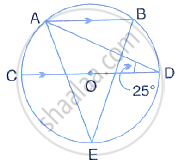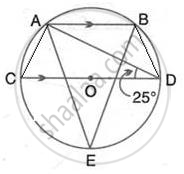Share

# In the Figure, Given Alongside, Ab ∥ Cd and O is the Centre of the Circle. If ∠Adc = 25°; Find the Angle Aeb Give Reasons in Support of Your Answer. - Mathematics

Course

#### Question

In the figure, given alongside, AB ∥ CD and O is the centre of the circle. If ∠ADC = 25°; find#### SolutionJoin AC and BD

∴ ∠CAD = 90° and ∠CBD = 90°
(Angle in a semicircle is a right angle) Also, AB || CD
∴ ∠ADB = 180°- 25° - BAC = 180° - 25° -115° = 40°
(pair of opposite angles in a cyclic quadrilateral are supplementary) Also, ∠AEB = ∠ADB = 40°
(Angle subtended by the same chord on the circle are equal)

Is there an error in this question or solution?